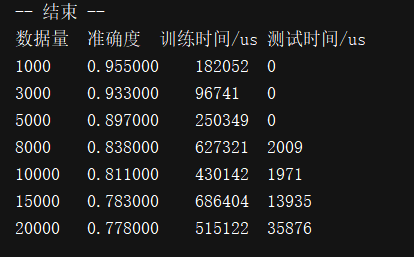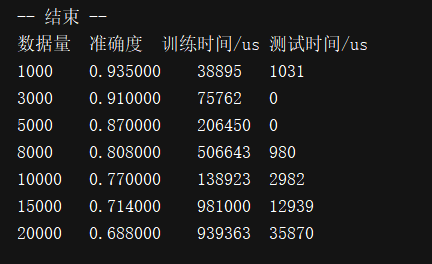# 2、效果图# 3、本次实验整体流程

1、先把整体样本读到内存中

2、把整体样本按照8：2的比例，分为80%的训练集，20%的测试集

3、然后“训练集”的样本 先分词，再转换为词向量

4、接着把训练集的样本和标签统一的传入算法中，得到拟合后的模型

5、把“测试集”的样本 先分词，再得到词向量

6、把测试集得出的词向量丢到拟合后的模型中，看得出的结果

7、把结果转换为准确率的形式，最后做成表格形式以便观看

# 4、这里用词向量，而不是TF-IDF预处理后的向量

TF-IDF（词频-逆文本频率），前面的TF也就是常说到的词频，我们之前做的向量化也就是做了文本中各个词的出现频率统计，并作为文本特征，这个很好理解。关键是后面的这个IDF，即“逆文本频率”如何理解。有些句子中的词，比如说“的”，几乎所有句子都会出现，词频虽然高，但是重要性却应该比 主语、宾语等低。IDF就是来帮助我们来反应这个词的重要性的，进而修正仅仅用词频表示的词特征值。vector_train = jiabaToVector(m_text_train, False, True)
...
...
vector_test = jiabaToVector(m_text_test, True, True)


# 5、源代码

import jieba
import datetime
# 向量\测试集\训练集\得分比对
from sklearn.model_selection  import train_test_split
from sklearn.feature_extraction.text import TfidfTransformer
from sklearn.feature_extraction.text import CountVectorizer
from sklearn.metrics import accuracy_score
#逻辑回归
from sklearn.linear_model import LogisticRegression

m_count = [1000,3000,5000,8000,10000,15000,20000]

# all
m_list_allText = []
m_list_allL4ID = []
# 内容的训练集、测试集
m_text_test = []
m_text_train = []
m_label_test = []
m_label_train = []

m_map_all = []

# 读取文件里面数据,获取标签和内容
def getFile(filename, count):
with open(filename, 'r' ,encoding='utf-8') as fp:
global m_list_allL4ID,m_list_allText
m_list_allL4ID = []
m_list_allText = []
for i in range(count):
if ":" in text:
L4ID = text.split(":")[-2]
Msg = text.split(":")[-1]
m_list_allL4ID.append(L4ID)
m_list_allText.append(Msg)

# 随机分为 测试集 和 训练集 2-8分
def randomTestAndTrain():
# 生成训练集和测试集
global m_text_test, m_text_train, m_label_test, m_label_train
m_text_train, m_text_test, m_label_train, m_label_test = train_test_split(m_list_allText, m_list_allL4ID, test_size=0.2, random_state=1)

def jiabaToVector(list, isTest, isTFIDF = False):
tmp_list = []
for sentence in list:
tmp_list.append(" ".join(jieba.cut(sentence.strip())))
# 利用TFIDF生成词向量
transformer = TfidfTransformer()
if isTest:
if isTFIDF:
tfidf = transformer.fit_transform(vectorizer.transform(tmp_list))
else:
tfidf = vectorizer.transform(tmp_list)
else:
if isTFIDF:
tfidf = transformer.fit_transform(vectorizer.fit_transform(tmp_list))
else:
tfidf = vectorizer.fit_transform(tmp_list)
return tfidf

# 创建默认参数的逻辑回归
def predict_4(X, Y):
lr =  LogisticRegression()
lr = lr.fit(X, Y)
return lr

def test(count):
# getFile("./rg_test.train", count)
getFile("./rg_train_20190102_20181227114134.train", count)
# print("获取全部已知数据的label和text")

# 随机分为 测试集 和 训练集 2-8分
randomTestAndTrain()

global vectorizer
# 全局向量
vectorizer = CountVectorizer()

# 生成训练向量
vector_train = jiabaToVector(m_text_train, False, False)

# 数据大小
lenall = len(m_list_allText)
# print("总集大小:", lenall)
print("总集大小:", lenall)

# 训练
startT_Train = datetime.datetime.now()
clf = predict_4(vector_train, m_label_train)
endT_Train = datetime.datetime.now()
print("训练Time:", (endT_Train - startT_Train).microseconds)

# 生成测试向量
vector_test = jiabaToVector(m_text_test, True, False)

# 测试
startT = datetime.datetime.now()
result = clf.predict(vector_test)
endT = datetime.datetime.now()
print("测试Time:", (endT - startT).microseconds)

# 计算百分比
percent = accuracy_score(result, m_label_test)
print("准确率:", round(percent, 3))

map_all = {}
map_all["精确率"]=round(percent, 3)
map_all["数据量"]=lenall
map_all["训练时间/us"]=(endT_Train - startT_Train).microseconds
map_all["测试时间/us"]=(endT - startT).microseconds
m_map_all.append(map_all)

if __name__ =="__main__":
print ("-- 开始 --")
for testC in m_count:
test(testC)
print ("-- 结束 --")

# 打印表格
print("数据量\t准确度\t训练时间/us\t测试时间/us")
for key in m_map_all:
print("%d\t%f\t%d\t%d"%(key["数据量"],key["精确率"],key["训练时间/us"],key["测试时间/us"]))



# 6、知识点普及

## 6.1逻辑回归优点

1）预测结果是界于0和1之间的概率；

2）可以适用于连续性和类别性自变量；

3）容易使用和解释；

## 6.2逻辑回归缺点

1）对模型中自变量多重共线性较为敏感，例如两个高度相关自变量同时放入模型，可能导致较弱的一个自变量回归符号不符合预期，符号被扭转。​需要利用因子分析或者变量聚类分析等手段来选择代表性的自变量，以减少候选变量之间的相关性；

2）预测结果呈“S”型，因此从log(odds)向概率转化的过程是非线性的，在两端随着​log(odds)值的变化，概率变化很小，边际值太小，slope太小，而中间概率的变化很大，很敏感。 导致很多区间的变量变化对目标概率的影响没有区分度，无法确定阀值。

04-151万+

#### Python实现逻辑回归（LogisticRegression）完整过程

04-207507

#### logistic回归详解11-291317

#### [TensorFlow深度学习入门]实战四·逻辑回归鸢尾花进行分类（对比均方根误差与softmax交叉熵误差区别）

10-101336

#### python sklearn-04：逻辑回归及其效果评估

06-081295

#### 机器学习算法之Logistic regression (逻辑回归)详解（一）

05-017万+

#### 逻辑回归（Logistic regression）详解-并用scikit-learn训练逻辑回归拟合Iris数据集

10-072408

#### LogisticRegression模型参数整理

08-09277

#### 机器学习之逻辑回归(Logistic Regression)

09-04330

#### 统计学习方法笔记(八)-逻辑斯谛回归(LogisticRegression)原理及python实现

08-144万+

#### 机器学习算法（一）：逻辑回归模型（Logistic Regression, LR）

03-249866

#### spark pipeline原理学习和记录

03-021552

#### 机器学习算法与Python实践之（七）逻辑回归（Logistic Regression）

03-13664

#### Tensorflow计算正确率、精确率、召回率

08-161万+

#### 【机器学习】逻辑斯蒂回归（Logistic Regression）详解

04-29807

#### 简单易懂的分类准确率（accuracy_score）

08-162575

#### 机器学习之逻辑回归（Logistic Regression）与决策边界详解与实现

01-111万+

#### 机器学习 之 决策树（Decision Tree）文本算法的精确率

04-02187

#### 逻辑回归模型（Logistic）实战应用——文本分类©️2020 CSDN 皮肤主题: 代码科技 设计师: Amelia_0503点击重新获取扫码支付1.余额是钱包充值的虚拟货币，按照1:1的比例进行支付金额的抵扣。
2.余额无法直接购买下载，可以购买VIP、C币套餐、付费专栏及课程。余额充值